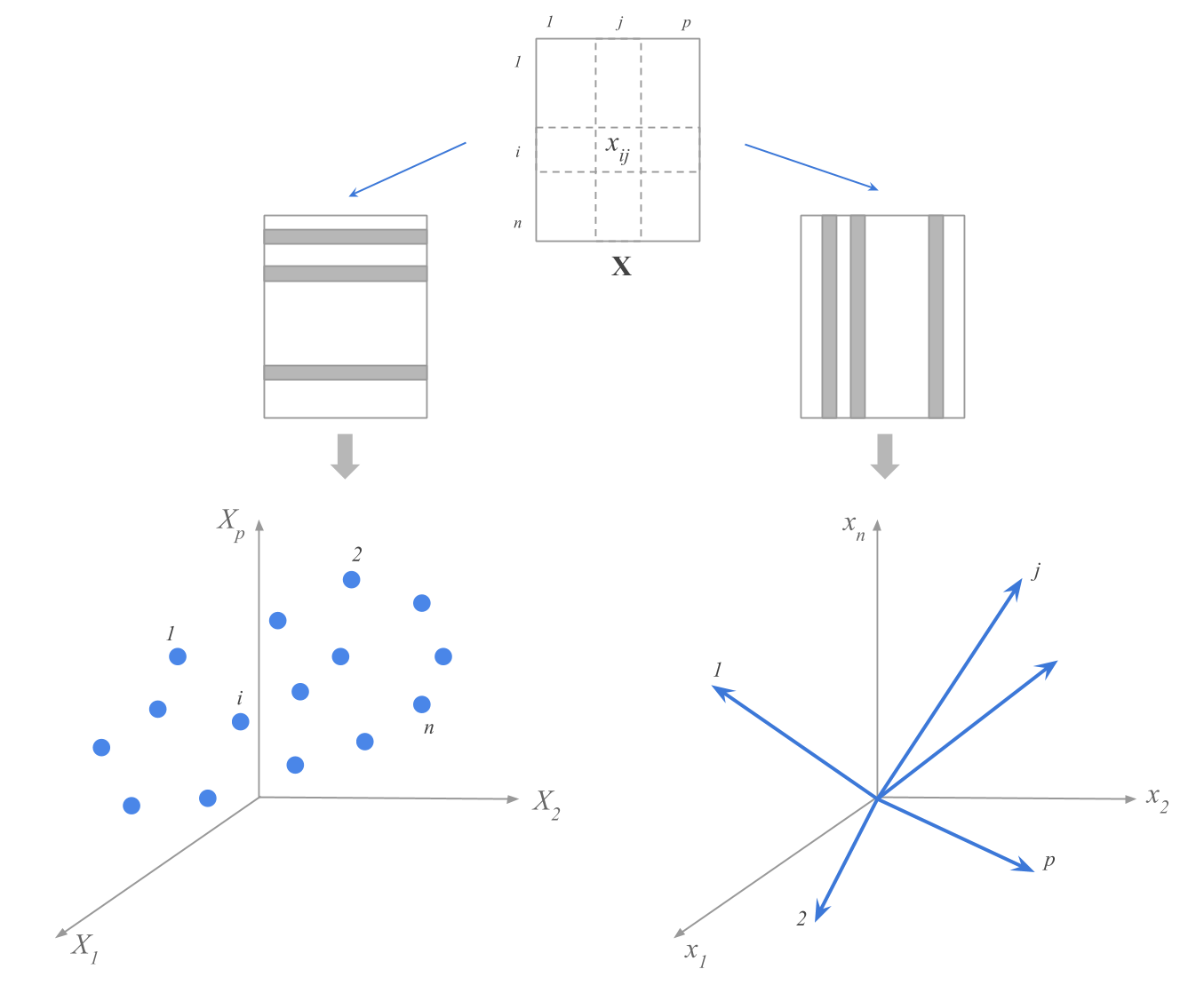# WelcomeThe purpose of this book is to help you understand how statistical notions are connected to matrix algebra concepts that constantly appear around Statistical Learning methods.

This book will teach you how to make the transition from a data table to a data matrix, how to think of a data matrix from a geometric perspective, and how to express—and interpret—statistical operations with vector-matrix notation. More specifically, you will learn how to express common statistical summaries (e.g. mean, variance, covariance, correlation) with vector-matrix notation. You will also learn how to see data—geometrically speaking—and how to provide a geometric interpretation to statistical summaries and related measures.

How to cite this book:

Sanchez, G. (2018) A Matrix Algebra Companion for Statistical Learning
https://www.gastonsanchez.com/matrix4sl

### Give Now

If you find this resource useful (which I’m pretty sure you will) please consider making a one-time donation in any amount. Your support really matters.